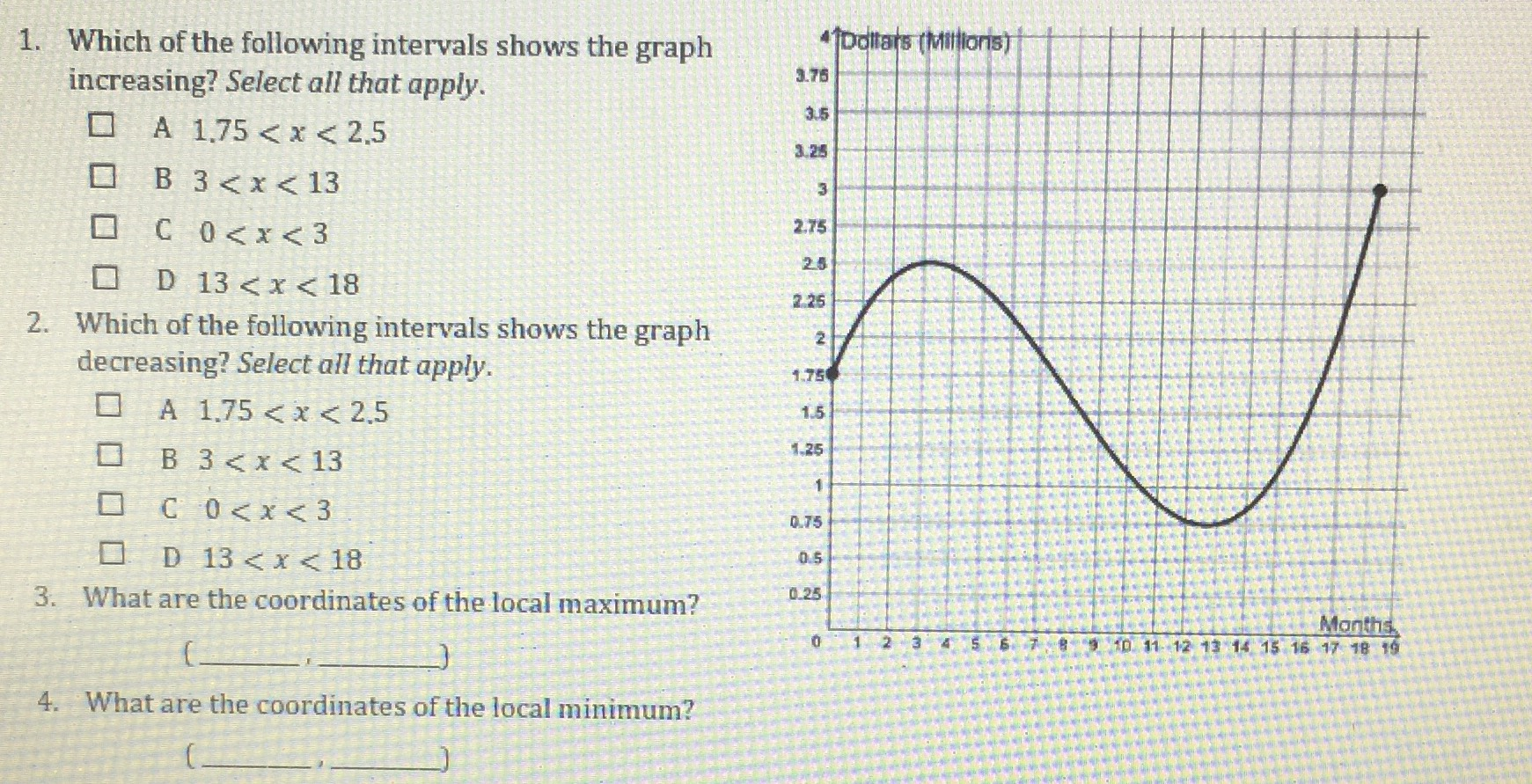### ¿Todavía tienes preguntas de matemáticas?

Pregunte a nuestros tutores expertos
Algebra
Pregunta1. Which of the following intervals shows the graph increasing? Select all that apply.

$$\square$$ A $$1.75 < x < 2.5$$

$$\square$$ B $$3 < x < 13$$

$$\square$$ C $$0 < x < 3$$

$$\square$$ D $$13 < x < 18$$

2. Which of the following intervals shows the graph decreasing? Select all that apply.

$$\square$$ A $$1.75 < x < 2.5$$

$$\square$$ B $$3 < x < 13$$

$$\square$$ C $$0 < x < 3$$

$$\square$$ D $$13 < x < 18$$

3. What are the coordinates of the local maximum?

4. What are the coordinates of the local minimum?